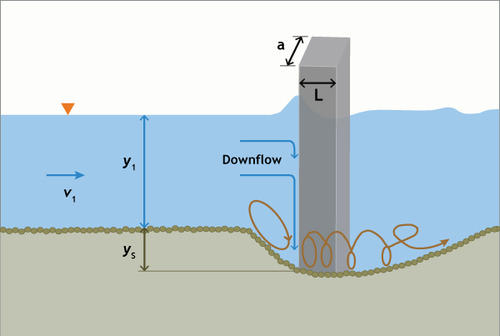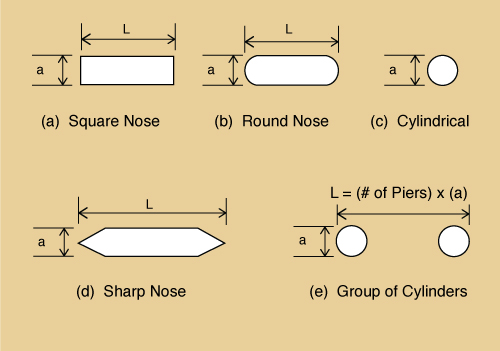online_scour_hec18:  Calculation of bridge scour using the HEC-18 formulaFormula:

Fr1 = v1/(g y1)1/2

ys /y1 = 2 K1 K2 K3 (a / y1)0.65 Fr10.43

[ SI Units (metric):  ys, y1, a, and L in m ]
[ U.S. Customary Units:  ys, y1, a, and L in ft ]
[ Fr = Froude number; g = gravitational acceleration ]

Reference:

DESCRIPTION      [Sample Input]     [Main page]

To determine the bridge scour depth ys, the calculator requires the following information:

• Flow depth directly upstream of the pier y1, in m (or ft).

• Mean flow velocity directly upstream of the pier v1, in m/s (or fps).

• Pier width in a horizontal direction normal to the flow a (Fig. 1), in m (or ft).Fig. 1  Definition sketch for pier scour.

• Pier length L (Fig. 1), in m (or ft).

• Angle of attack θ of the flow, in degrees, to determine the correction factor K2.

• Pier nose shape (Fig. 2), to determine the correction factor K1.Fig. 2  Common pier shapes.

• The bed condition (a choice between clear-water, plane bed and antidune flow, small dunes, medium dunes, and large dunes), to determine the correction factor K3.